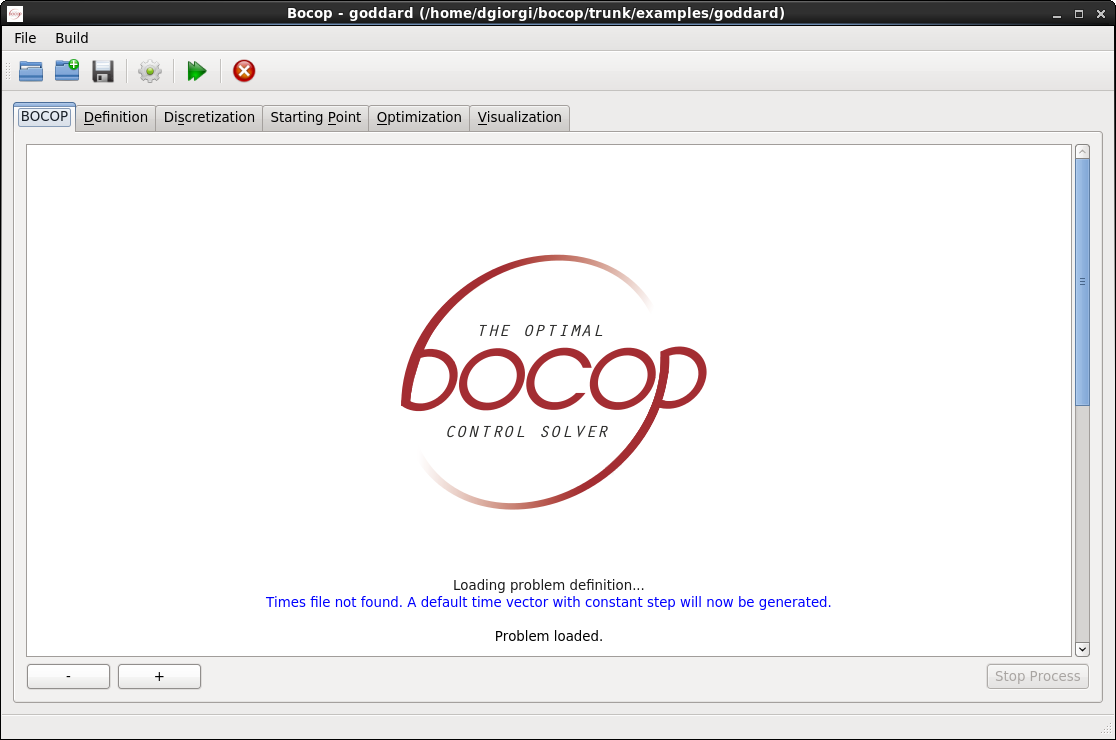# Features

The Bocop project aims to develop an open-source toolbox for solving optimal control problems, with collaborations with industrial and academic partners.

Optimal control (optimization of dynamical systems governed by differential equations) has numerous applications in transportation, energy, process optimization, and biology.

Bocop is developed since 2010 in the framework of the Inria-Saclay initiative for an open source optimal control toolbox, and is supported by the team Commands.

Since 2014 a second package, called BocopHJB, using a Dynamic Programing approach is developed.

Bocop is released under the Eclipse Public License (EPL).

Contact: Pierre Martinon

The original Bocop package implements a local optimization method. The optimal control problem is approximated by a finite dimensional optimization problem (NLP) using a time discretization (the direct transcription approach). The NLP problem is solved by the well known software Ipopt, using sparse exact derivatives computed by ADOL-C.

Key features

• User friendly and advanced GUI for efficient use
• Uses the famous Ipopt solver for the discretized NLP problem
• Sparse derivatives computed automatically by Adol-C / ColPack
• Open-source package, highly modularized architecture
• Built-in parameter estimation module

View of the main interface (Qt)The second package BocopHJB implements a global optimization method. Similarly to the Dynamic Programming approach, the optimal control problem is solved in two steps. First we solve the Hamilton-Jacobi-Bellman equation satisfied by the value function of the problem. Then we simulate the optimal trajectory from any chosen initial condition. The computational effort is essentially taken by the first step, whose result, the value function, can be stored for subsequent trajectory simulations.

Key features

• Global optimization for both deterministic and stochastic optimal control problems
• Handles switching between discrete modes of the system
• Stopping time problems can be solved using switchings
• Built-in simulation module to recompute optimal strategies
• Support advanced rules to define the discrete control set
• Parallel execution with OpenMP
• Matlab/Python scripts to read value function and simulated trajectories